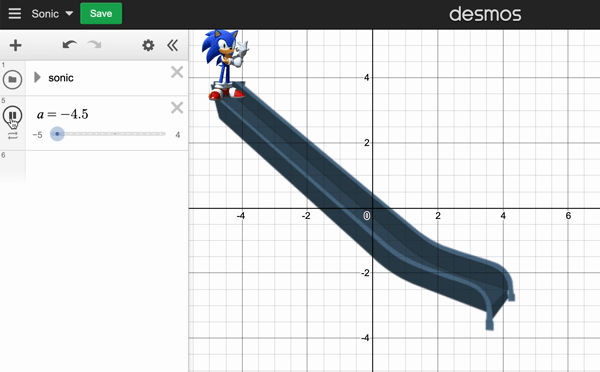top of page# Math Fan Content

## << Lessons

###### TASK #1 Slides with Desmos

When Sonic the Hedgehog (35 kg (77 lb.) came to Earth, he slide down a slide of the length of 12.8 meters and the height of 8 meters without using his enormous speed and comes to a gradual stop at the bottom. Calculate the energy transferred and average frictional force on Sonic.Just before Sonic starts sliding, he has a gravitational potential energy which  first turns to kinetic energy since he slides. We also know that he gradually stops and that's because of the friction ( slide's surface and air resistance). When he stopped, we can roughly say that his kinetic energy  transformed the thermal energy.

What is the steepness of the slide? What happens if we increase the length of the slide without changing its height?

If we increase the height of the slide to 10 meters, how long a slide we need to make him gradually stop without falling off the slide.

Calculations behind the Slope slides can be more complex. Here is a manufacturer's website about the measurements.###### TASK #2 Swing - outdoor

Note the mass of the person who will be on the swing in kilograms.

Measure the length (the length of the ropes or chains) of the swing.

Estimate how high the person can swing using a meter stick.

Calculate the potential energy of the person at this height.

(g =10 N/kg)

EP = mgh

Estimate the angle of a complete back and forth swing.

Find the distance of the kid travel using the angle and the length of the swing.

Record the time it takes for the swing to go from one side to the other side.

Calculate the angular velocity of the swinger.

Now Calculate the kinetic energy of the swinger

EK = ½ mv2

Here is a Desmos lesson about Modelling the Motion of a Swing.Yoda (40kg) and Anakin (90 kg) want to  bring balance to the force. They are on a 2 meters long seesaw. Where should they place the pivot point to create the balance?###### TASK #3b Seesaw with a fixed pivot

Yoda (40kg) and Anakin (90 kg) want to  bring balance to the force. They are on a seesaw with a fixed pivot. Force (and the Polypad) gives them the power to clone themselves as many as they wish. Create the balance on the Polypad by cloning them.###### TASK #4 Measuring the height of trees - Clinometer Activity

Find a place to stand where you can see the top of the tree. Keep your distance from the tree measurable and as big as possible.

You can easily make a clinometer using a large protractor, a straw.  Check the for detailed instructions.

Look through the clinometer to see the top of the tree. Read the angle on the clinometer.

Here you created a right triangle where you know an angle and a side (distance from the tree). With the help of trigonometry, you can calculate the height of the tree.

bottom of page